### Moment of inertia and CM

Center of mass

#### Problem:

A uniform carpenter's square has the shape of an L, as shown in the figure.  Locate the center of mass relative to the origin of the coordinate system.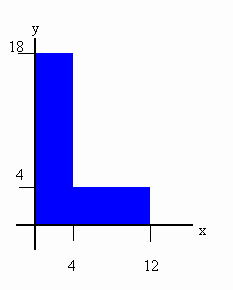Solution:

• Concepts:
The center of mass
• Reasoning:
We are asked to find the CM of an object.
We can think of the system as being made up of two subsystems, as shown in the figure.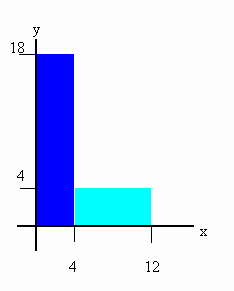The CM of the left subsystem lies at xCM = 2 cm, yCM = 9 cm.  The CM of the right subsystem lies at xCM = 8 cm, yCM = 2 cm.  If the mass of a 1 cm by 1 cm square is 1 unit, then the mass of the left subsystem is 72 units and the mass of the right subsystem is 32 units.  We find the CM of the system by treating each subsystem as a separate particle, with all its mass concentrated at its center of mass.

• Details of the calculation:
xCM = (72 units 2 cm + 32 units 8 cm)/(104 units) = 3.85 cm,
yCM = (72 units 9 cm + 32 units 2 cm)/(104units) = 6.85 cm.
The CM of the system lies outside of the system.  For irregular-shaped objects it is quite common for the CM to lie outside the system.  This special point outside the system responds to external forces as if the total mass of the system were concentrated there.

Moment of inertia

#### Problem:

The four particles in the figure below are connected by rigid rods.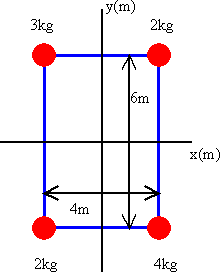The origin is at the center of the rectangle.  If the system rotates in the x-y plane about the z-axis with an angular speed of 6 rad/s, calculate
(a) the moment of inertia of the system about the z-axis and
(b) the rotational energy of the system.

Solution:

• Concepts:
The moment of inertia about an axis, the rotational kinetic energy
• Reasoning:
The moment of inertia is I = ∑miri2.  Here ri is the perpendicular distance of particle i from the z-axis.
the rotational kinetic energy, Trot = ½Iω2
• Details of the calculation:
Each particle is a distance r = (9 + 4)½ m = √(13) m from the axis of rotation.
I = (3 kg + 2 kg + 4 kg + 2 kg)(13 m2) = 1 43 kgm2.
(b) The rotational kinetic energy is Trot = ½Iω2 = 71.5*36/s2 = 2574 J.

#### Problem:

Three particles are connected by rigid rods of negligible mass lying along the y-axis as shown.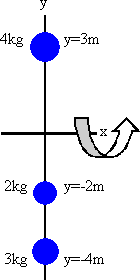If the system rotates about the x-axis with angular speed of 2 rad/s, find
(a)  the moment of inertia about the x-axis and the total rotational kinetic energy evaluated from ½Iω2, and
(b)  the linear speed of each particle and the total kinetic energy evaluated from ∑½mivi2.

Solution:

• Concepts:
The moment of inertia of a system about an axis
• Reasoning:
The moment of inertia is I = ∑miri2
Here ri is the perpendicular distance of particle i from the x-axis.
• Details of the calculations:
(a)  I = 4 kg 9 m2 + 2 kg 4 m2 + 3 kg 16 m2 = 92 kgm2.
The rotational kinetic energy is Trot = ½Iω2 = 46*4/s2 = 184 J.
(b)  The linear speed of particle i is vi = ωri.
The linear speed of the 4 kg mass is v = 6 m/s, and its kinetic energy is ½mv2 = 72 J.
The linear speed of the 2 kg mass is v = 4 m/s, and its kinetic energy is ½mv2 = 16 J.
The linear speed of the 3 kg mass is v = 8 m/s, and its kinetic energy is ½mv2 = 96 J.
The sum of the kinetic energies of the three particles is 184 J.

#### Problem:

Two circular metal disks have the same mass M and the same thickness d.  Disk 1 has a uniform density ρ1 which is less than ρ2, the uniform density of disk 2.  Which disk, if either, has the larger moment of inertia about its symmetry axis perpendicular to the plane of the disk?

Solution:

• Concepts:
The moment of inertia
• Reasoning:
Let the radii of the disks be R1 and R2 respectively.
The disks have the same mass and thickness.  Therefore ρ1R12 = ρ2R22.
The moments of inertia are I1 = MR12/2 and I2 = MR22/2.
• Details of the calculation:
I1/I2 = R12/R22 =  ρ21.
Since ρ1 < ρ2, I1 > I2.  Disk 1 has the larger moment of inertia.

#### Problem:

Find the fraction of the kinetic energy that is translational and rotational when
(a)  a hoop
(b)  a disc and
(c)  a sphere rolls down an inclined plane of height h.  Find the velocity at the bottom in each case.  Compare with a block sliding without friction down the plane.

#### Problem:

A uniform rectangular object of mass m with sides a and b (b > a) and negligible thickness rotates with constant angular velocity ω about a diagonal through the center.  Ignore gravity.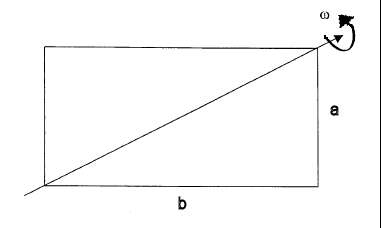(a)  What are the principal axes and principal moments of inertia?
(b)  What is the angular momentum vector in the body coordinate system?
(c)  What external torque must be applied to keep the object rotating with constant angular velocity about the diagonal?